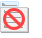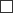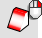# ThicknessAnalysisThe ThicknessAnalysis command uses false-color display to evaluate the thickness of a solid.

• The ThicknessAnalysis command is not designed to evaluate the distance between two independent surfaces. To evaluate the distance between two surfaces compare the points on the first surface to the second surface using the PointDeviation command.
• The easiest way to generate a set of points on a surface is to create a mesh using the Mesh command and then use PointDeviation on the resulting mesh object and the second surface.
##### How thickness is evaluated?

For every vertex in the analysis mesh the distance from a vertex to the "other side" of the mesh is calculated. A color is assigned to the vertex based on that distance. If the distance is less than or equal to the minimum distance the color is red. If the distance is greater than the maximum distance, the color is white. If the distance is between the minimum and maximum distances, a color between red and blue is assigned. Distance close to the minimum distance are more red than blue and distances close to the maximum distance are more blue than red. A report is printed in the command prompt window that lists the number of vertices tested and the percentage of vertices with distances less than or equal to the minimum distance, between the minimum and maximum distances, and beyond the maximum distance.

Command-line options

If the analysis needs to take a long time, a prompt to continue appears.

##### Yes

Continues the analysis for an additional six minutes.

##### No

Stops the analysis.

##### ForAsLongAsItTakes

Continues the analysis no matter how long it takes.

#### Warning

Once you commit to allowing the analysis to continue, you cannot stop the command with Esc.

Thickness Analysis options

##### Maximum / minimum distances

For every vertex in the analysis mesh the distance from a vertex to the "other side" of the mesh is calculated. A color is assigned to the vertex based on that distance. If the distance is less than or equal to the minimum distance the color is red. If the distance is greater than the maximum distance, the color is white. If the distance is between the minimum and maximum distances, a color between red and blue is assigned. Distance close to the minimum distance are more red than blue and distances close to the maximum distance are more blue than red. A report is printed in the command prompt window that lists the number of vertices tested and the percentage of vertices with distances less than or equal to the minimum distance, between the minimum and maximum distances, and beyond the maximum distance.

#####Show edges and isocurves

Display edges or isocurves on the analyzed objects.

Open Polygon Mesh Options to change analysis mesh density. Increasing mesh density improves the analyzing accuracy.

Select more objects to analyze.

##### Remove Objects

Remove selected objects from the analysis.

# ThicknessAnalysisOffThe ThicknessAnalysisOff command turns off thickness analysis display.